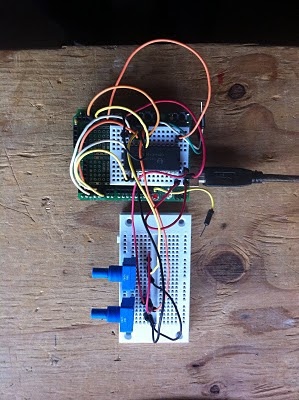## Saturday, March 5, 2011

### Analog Multiplexing

The Arduino has 6 analog I/O pins. For one project I'm considering, I actually will need 7 analog I/O pins, so I went looking for an analog multiplexer chip that would allow me to have more analog I/O. I found the CD74HC4067, a 16-channel analog multiplexer that will fill the bill.

You can think of the chip like a 16-position switch. There are 4 input pins that select which one of the 16 pins to connect to the common pin. If you write a binary 0000 to the input pins, the common pin is connected to input/output pin 0. If you write a binary 0001, the common pin is connected to input/output pin 1. And so on.

To try this chip out, I built the circuit shown below. Two 10 k potentiometers are wired up as a voltage divider to provide a voltage from 0-5 volts that I can measure. The common pin of the multiplexer chip goes to the Arduino's analog input, and the wipers of the pots go to input/output pins 0 and 1 of the multiplexer chip.To be sure I was programming the chip correctly, I also hooked the wipers of the pots to Arduino analog pins 1 and 2. If I've got things working properly, then I should see that same value coming from the mux chip as I see on the Arduino analog input.

Finally, I wrote a little sketch that selects one of the mux inputs, and then prints the values from analogRead() on pin 0 (connected to the MUX) and pins 1 and 2 (connected to the potentiometers). Running the sketch, when I turn the potentiometer selected by the mux, I see it value change, and it matches the value directly read by the Arduino. Unit testing hardware FTW!

`/**74HC4067 TestThe 74HC4067 chip is an analog multiplexer. It has 4 control pins where you writea value (0-15) that selects which of the 16 analog inputs/outputs to/from the chipare hooked up to the common input/outout. This is useful if you need to read orwrite an analog voltage to more than the 6 pins available on the Arduino.*/int mpin = 1;void setup() {  Serial.begin(9600);  pinMode(2, OUTPUT);  pinMode(3, OUTPUT);  pinMode(4, OUTPUT);  pinMode(5, OUTPUT);}/*Choose the input pin selected by the 74HC4067 chip*/void selectInputPin(int pin) { if (0 == pin) {   digitalWrite(2, 0); } else if (1 == pin) {   digitalWrite(2, 1); } digitalWrite(3, 0); digitalWrite(4, 0); digitalWrite(5, 0);}    void loop() {  Serial.print("Loop begin: select multiplexer pin ");  selectInputPin(mpin);  Serial.print(mpin);  Serial.println();  Serial.print("0: ");  Serial.print(analogRead(0));  Serial.print(" 1: ");  Serial.print(analogRead(1));  Serial.print(" 2: ");  Serial.print(analogRead(2));  Serial.println();  delay(1000);}`